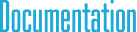#cySpatial.h File Reference

## Detailed Description

Spatial vector algebra classes.

This file includes spatial vector algebra classes intended for the implementation of Featherstone's articulated rigid body dynamics method. SpatialVector6 class is both for spatial motion vectors and spatial force vectors, SpatialTrans6 is a spatial matrix class for coordinate transformations only, and SpatialMatrix6 is the general spatial matrix class.

`#include "cyPoint.h"`
`#include "cyMatrix.h"`

## Classes

class  SpatialVector6< TYPE >

class  SpatialTrans6< TYPE >

class  SpatialMatrix6< TYPE >

## Typedefs

typedef SpatialVector6< float > SpatialVector6f

typedef SpatialTrans6< float > SpatialTrans6f

typedef SpatialMatrix6< float > SpatialMatrix6f

typedef SpatialVector6< double > SpatialVector6d

typedef SpatialTrans6< double > SpatialTrans6d

typedef SpatialMatrix6< double > SpatialMatrix6d

typedef cy::SpatialVector6f cySpatialVector6f

typedef cy::SpatialTrans6f cySpatialTrans6f

typedef cy::SpatialMatrix6f cySpatialMatrix6f

typedef cy::SpatialVector6d cySpatialVector6d

typedef cy::SpatialTrans6d cySpatialTrans6d

typedef cy::SpatialMatrix6d cySpatialMatrix6d

## Functions

template<typename TYPE >
SpatialMatrix6< TYPE > operator& (const SpatialVector6< TYPE > &v0, const SpatialVector6< TYPE > &v1)

## § SpatialVector6f

 typedef SpatialVector6 SpatialVector6f

6D spatial vector (for 3D) with float type elements

## § SpatialTrans6f

 typedef SpatialTrans6 SpatialTrans6f

6D spatial matrix for coordinate transforms with float type elements

## § SpatialMatrix6f

 typedef SpatialMatrix6 SpatialMatrix6f

6D spatial matrix with float type elements

## § SpatialVector6d

 typedef SpatialVector6 SpatialVector6d

6D spatial vector (for 3D) with double type elements

## § SpatialTrans6d

 typedef SpatialTrans6 SpatialTrans6d

6D spatial matrix for coordinate transforms with double type elements

## § SpatialMatrix6d

 typedef SpatialMatrix6 SpatialMatrix6d

6D spatial matrix with double type elements

## § cySpatialVector6f

 typedef cy::SpatialVector6f cySpatialVector6f

6D spatial vector (for 3D) with float type elements

## § cySpatialTrans6f

 typedef cy::SpatialTrans6f cySpatialTrans6f

6D spatial matrix for coordinate transforms with float type elements

## § cySpatialMatrix6f

 typedef cy::SpatialMatrix6f cySpatialMatrix6f

6D spatial matrix with float type elements

## § cySpatialVector6d

 typedef cy::SpatialVector6d cySpatialVector6d

6D spatial vector (for 3D) with double type elements

## § cySpatialTrans6d

 typedef cy::SpatialTrans6d cySpatialTrans6d

6D spatial matrix for coordinate transforms with double type elements

## § cySpatialMatrix6d

 typedef cy::SpatialMatrix6d cySpatialMatrix6d

6D spatial matrix with double type elements

## § operator&()

 SpatialMatrix6 cy::operator & ( const SpatialVector6< TYPE > & v0, const SpatialVector6< TYPE > & v1 )

tensor product (outer product) of two vectors